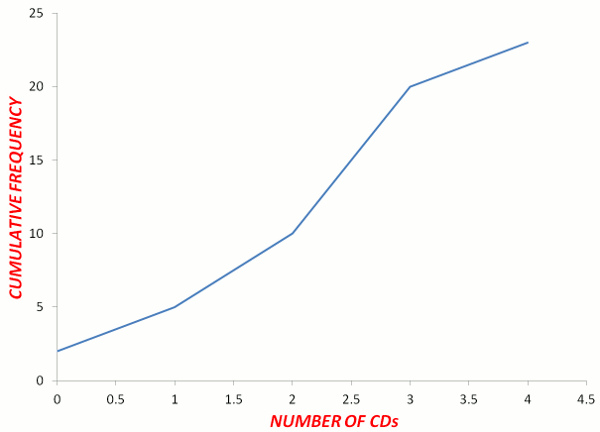# Ogives (Cumulative Frequency Graphs)

Before viewing this page, it would be helpful to learn how to calculate the Median and Quartiles.

Cumulative Frequency is the progressive total of the frequencies. It assists us to find the median, quartiles and percentiles from large quantities of data organized into tables and graphs. To find the cumulative frequency, you add up the frequencies row by row.

An Ogive is a graph of the cumulative frequency. Interpreting quartiles, median and percentiles from a graph is more accurate than from a table.

## Example - Teenagers' CD CollectionsThe following table shows the number of music CDs owned by students in a Math class. Complete the table with two more columns for the cumulative frequency and cumulative percentage. Draw an ogive (a cumulative frequency graph). From the ogive, find the 1st quartile, median, 3rd quartile and 80th percentile.

 NUMBER OF CDs NUMBER OF TEENAGERS 0 2 1 3 2 5 3 10 4 5

Answer:

 NUMBER OF CDs NUMBER OF STUDENTS CUMULATIVE FREQUENCY CUMULATIVE PERCENTAGE 0 2 2 2⁄23 × 100 = 8.7% 1 3 5 5⁄23 × 100 = 21.7% 2 5 10 10⁄23 × 100 = 43.5% 3 10 20 20⁄23 × 100 = 87% 4 3 23 23⁄23 × 100 = 100% Σ f = 23From Table:

n = 25

 1st Quartile = (n + 1) ÷ 4 = (23 + 1) ÷ 4 = 6th student = 2 CDs Median = (n + 1) ÷ 2 = (23 + 1) ÷ 2 = 12th student = 3 CDs 3rd Quartile = (n + 1) ÷ 4 × 3 = (23 + 1) ÷ 4 × 3 = 18th student = 3 CDs 80th percentile = 80% of 25 students = 20th student = 3 CDs

From Graph (more accurate):

 1st Quartile = (n + 1) ÷ 4 = (23 + 1) ÷ 4 = 6th student = 1.2 CDs Median = (n + 1) ÷ 2 = (23 + 1) ÷ 2 = 12th student = 2.2 CDs 3rd Quartile = (n + 1) ÷ 4 × 3 = (23 + 1) ÷ 4 × 3 = 18th student = 2.8 CDs 80th percentile = 80% of 25 students = 20th student = 3.0 CDs

## Did You Know That...?

Michael Jackson's "Thriller" album with sales of 110 million is the best-selling album of all time.